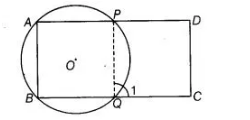# ABCD is a parallelogram. A circle through A,`
Question:

ABCD is a parallelogram. A circle through A, B is so drawn that it intersects AD at P and BC at Q. Prove that P, Q, C and D are concyclic.

Solution:

Given ABCD is a parallelogram. A circle whose centre O passes through A, B is so drawn that it intersect AD at P and BC at Q To prove Points P, Q, C and D are con-cyclic.Construction Join PQ

Proof ∠1 = ∠A [exterior angle property of cyclic quadrilateral]

But  ∠A = ∠C [opposite angles of a parallelogram]

∴ ∠1 = ∠C ,..(i)

But ∠C+ ∠D = 180°  [sum of cointerior angles on same side is 180°]

=> ∠1+ ∠D = 180° [from Eq. (i)]

Thus, the quadrilateral QCDP is cyclic.

So, the points P, Q, C and D are con-cyclic. Hence proved.